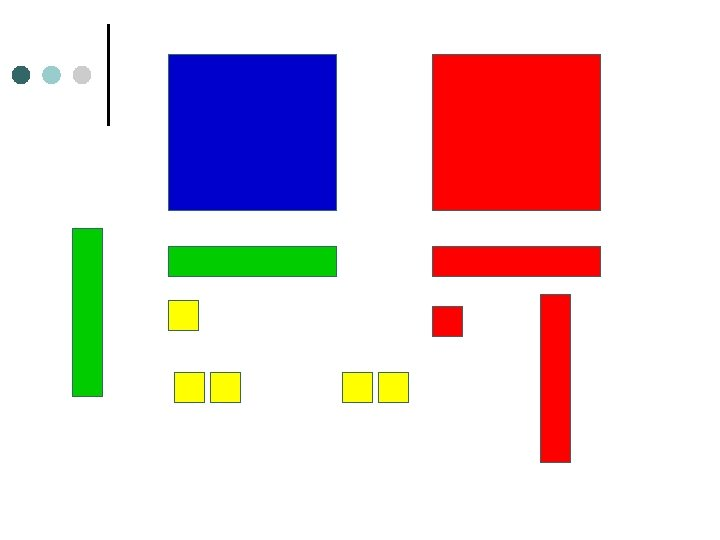Скачать презентацию Let s Do Algebra Tiles David Mc Reynolds AIMS

14ba1fa39f4c0ebc08e30c7a0cea4a02.ppt

• Количество слайдов: 56Let’s Do Algebra Tiles David Mc. Reynolds AIMS Pre. K-16 Project Noel Villarreal South Texas Rural Systemic Initiative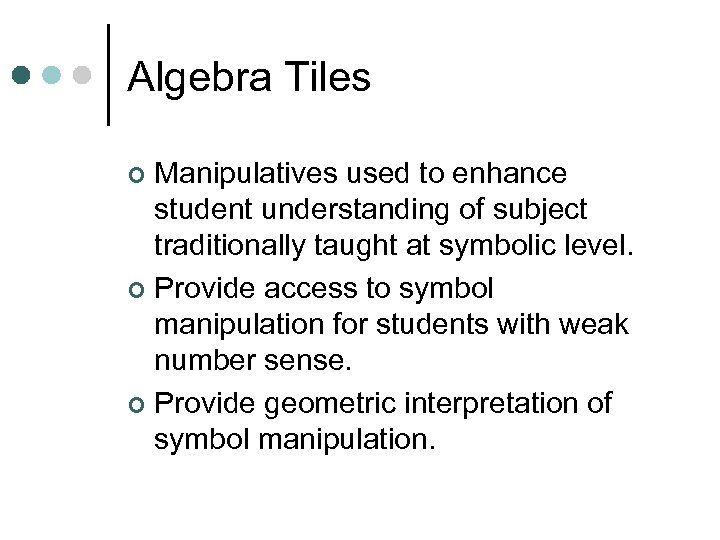Algebra Tiles Manipulatives used to enhance student understanding of subject traditionally taught at symbolic level. ¢ Provide access to symbol manipulation for students with weak number sense. ¢ Provide geometric interpretation of symbol manipulation. ¢Algebra Tiles Support cooperative learning, improve discourse in classroom by giving students objects to think with and talk about. ¢ When I listen, I hear. ¢ When I see, I remember. ¢ But when I do, I understand. ¢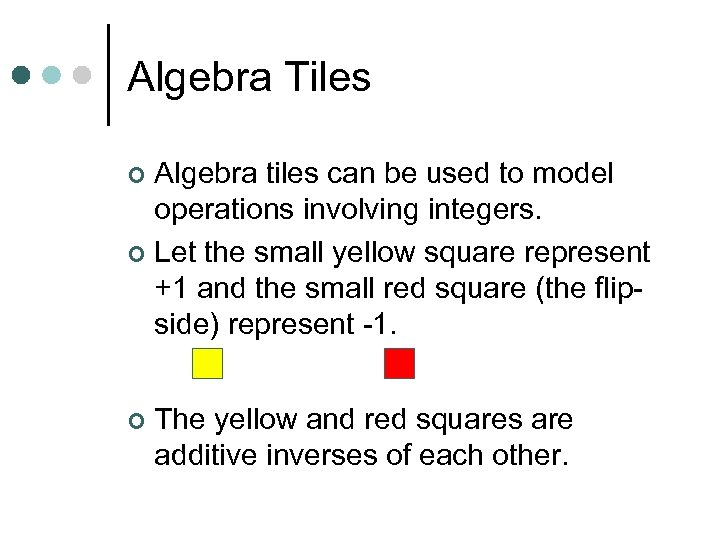Algebra Tiles Algebra tiles can be used to model operations involving integers. ¢ Let the small yellow square represent +1 and the small red square (the flipside) represent -1. ¢ ¢ The yellow and red squares are additive inverses of each other.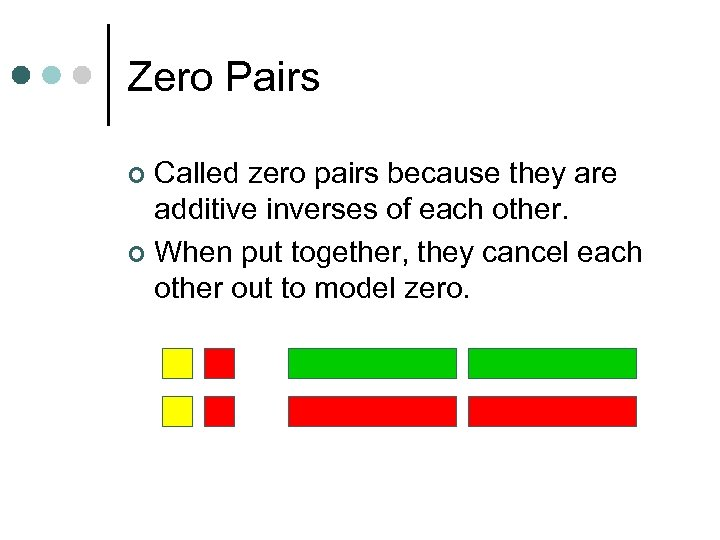Zero Pairs Called zero pairs because they are additive inverses of each other. ¢ When put together, they cancel each other out to model zero. ¢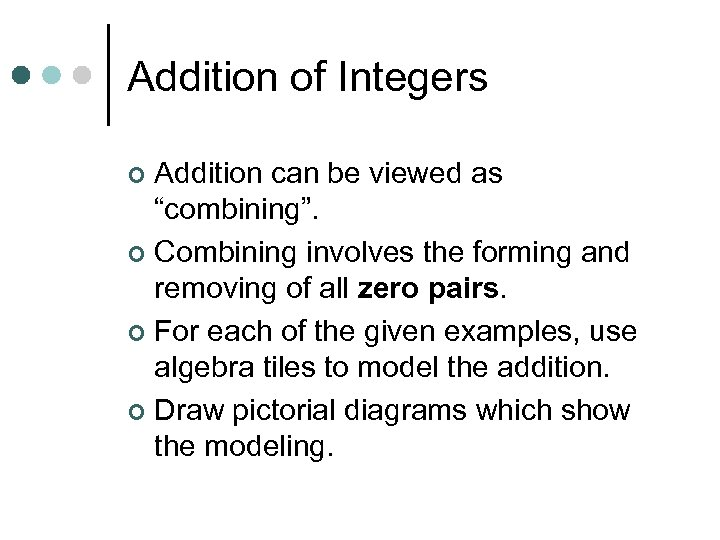Addition of Integers Addition can be viewed as “combining”. ¢ Combining involves the forming and removing of all zero pairs. ¢ For each of the given examples, use algebra tiles to model the addition. ¢ Draw pictorial diagrams which show the modeling. ¢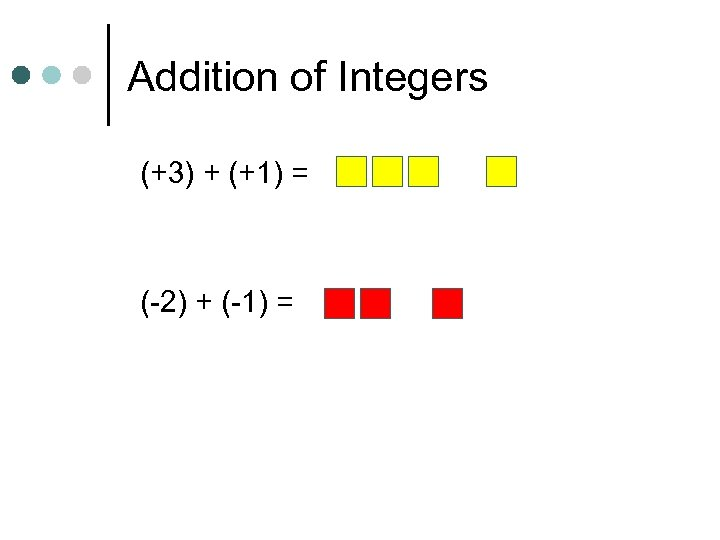Addition of Integers (+3) + (+1) = (-2) + (-1) =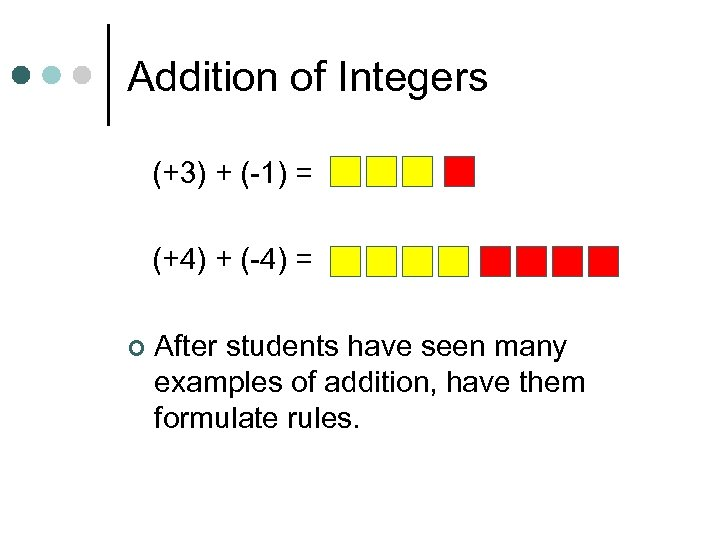Addition of Integers (+3) + (-1) = (+4) + (-4) = ¢ After students have seen many examples of addition, have them formulate rules.Subtraction of Integers Subtraction can be interpreted as “take-away. ” ¢ Subtraction can also be thought of as “adding the opposite. ” ¢ For each of the given examples, use algebra tiles to model the subtraction. ¢ Draw pictorial diagrams which show the modeling process. ¢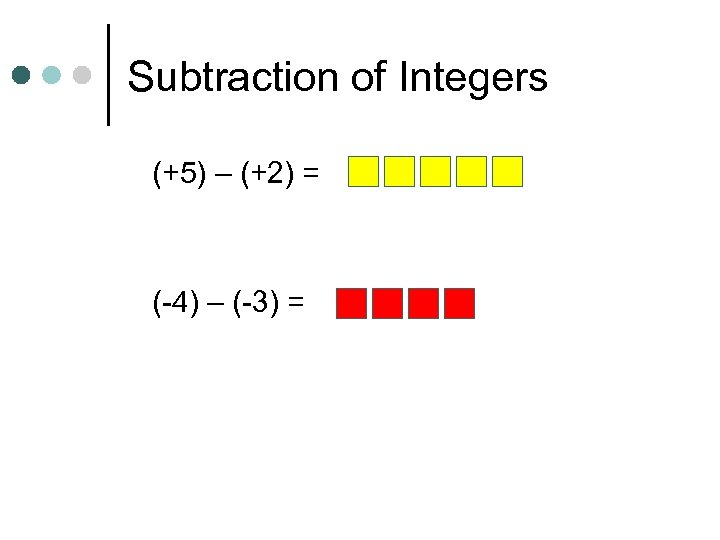Subtraction of Integers (+5) – (+2) = (-4) – (-3) =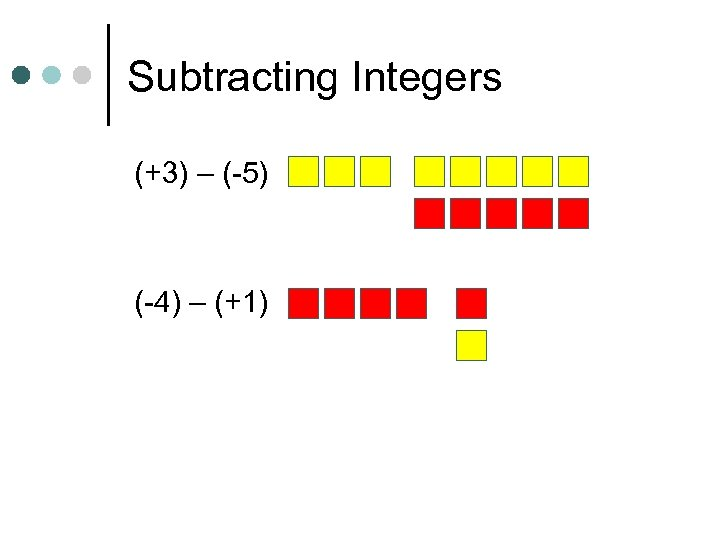Subtracting Integers (+3) – (-5) (-4) – (+1)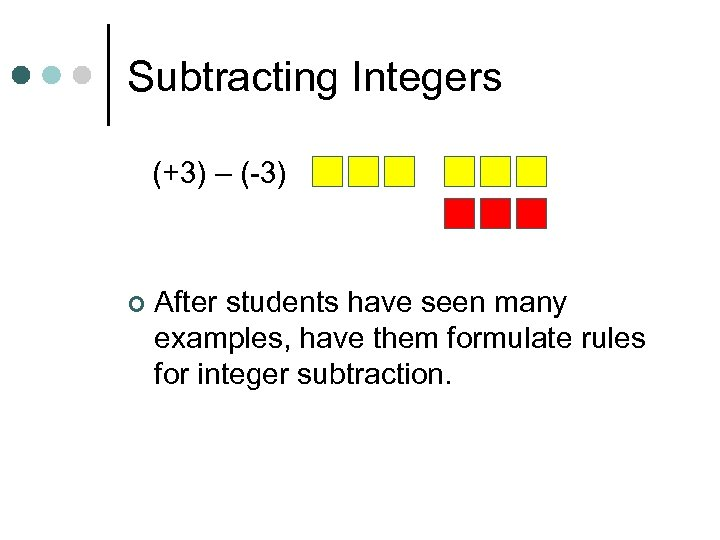Subtracting Integers (+3) – (-3) ¢ After students have seen many examples, have them formulate rules for integer subtraction.Multiplication of Integers ¢ ¢ Integer multiplication builds on whole number multiplication. Use concept that the multiplier serves as the “counter” of sets needed. For the given examples, use the algebra tiles to model the multiplication. Identify the multiplier or counter. Draw pictorial diagrams which model the multiplication process.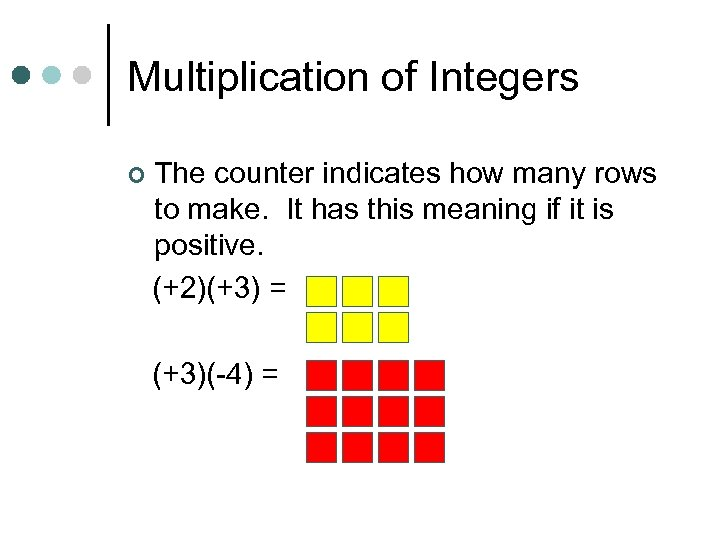Multiplication of Integers ¢ The counter indicates how many rows to make. It has this meaning if it is positive. (+2)(+3) = (+3)(-4) =Multiplication of Integers ¢ If the counter is negative it will mean “take the opposite of. ” (flip-over) (-2)(+3) (-3)(-1)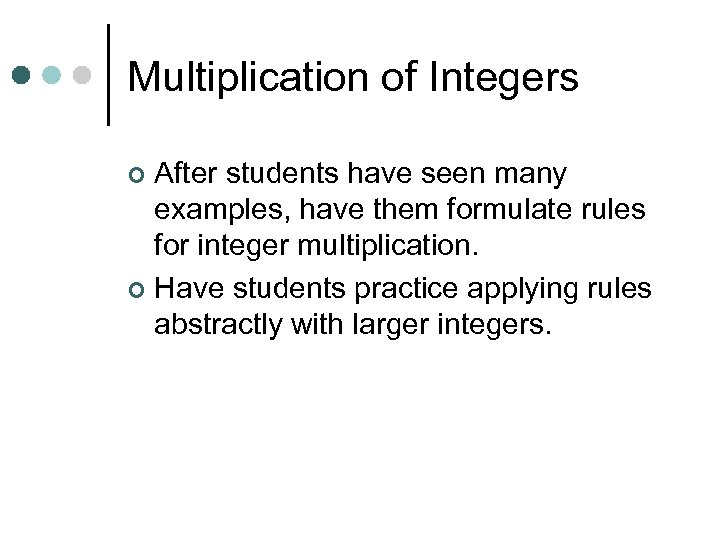Multiplication of Integers After students have seen many examples, have them formulate rules for integer multiplication. ¢ Have students practice applying rules abstractly with larger integers. ¢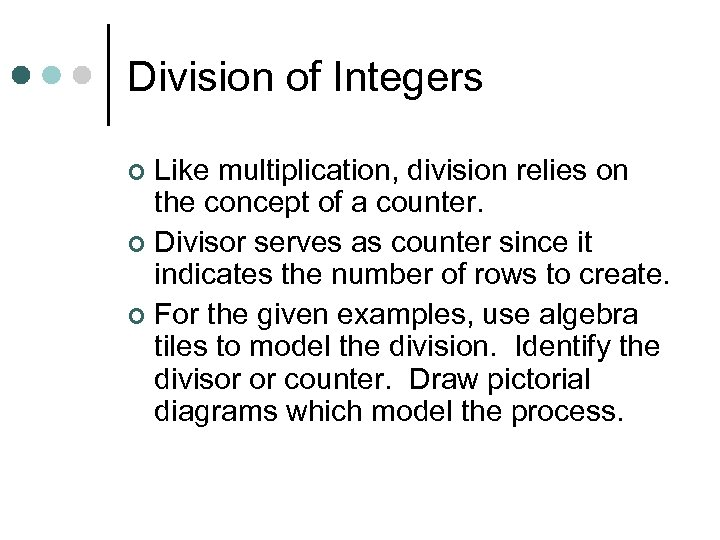Division of Integers Like multiplication, division relies on the concept of a counter. ¢ Divisor serves as counter since it indicates the number of rows to create. ¢ For the given examples, use algebra tiles to model the division. Identify the divisor or counter. Draw pictorial diagrams which model the process. ¢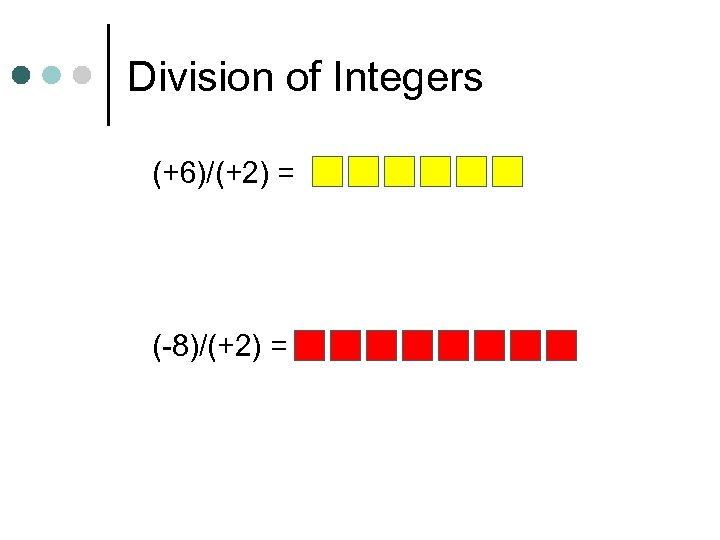Division of Integers (+6)/(+2) = (-8)/(+2) =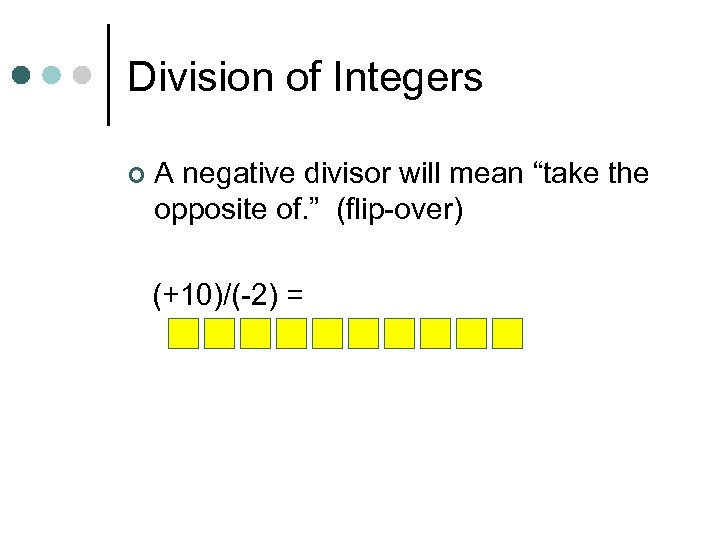Division of Integers ¢ A negative divisor will mean “take the opposite of. ” (flip-over) (+10)/(-2) =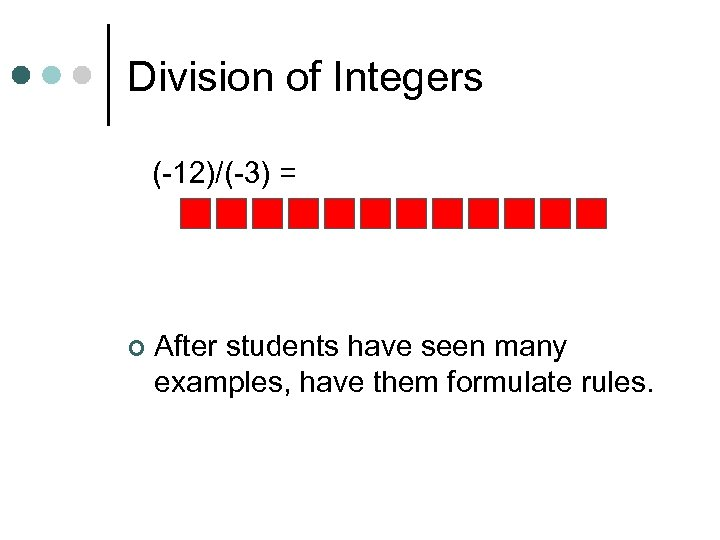Division of Integers (-12)/(-3) = ¢ After students have seen many examples, have them formulate rules.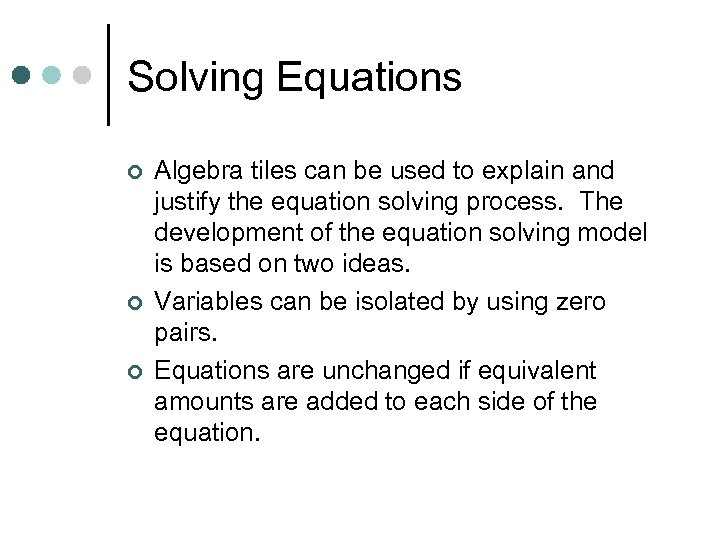Solving Equations ¢ ¢ ¢ Algebra tiles can be used to explain and justify the equation solving process. The development of the equation solving model is based on two ideas. Variables can be isolated by using zero pairs. Equations are unchanged if equivalent amounts are added to each side of the equation.Solving Equations ¢ Use the green rectangle as X and the red rectangle (flip-side) as –X (the opposite of X). X+2=3 2 X – 4 = 8 2 X + 3 = X – 5Solving Equations X+2=3 2 X – 4 = 8Solving Equations 2 X + 3 = X – 5Distributive Property Use the same concept that was applied with multiplication of integers, think of the first factor as the counter. ¢ The same rules apply. 3(X+2) ¢ Three is the counter, so we need three rows of (X+2) ¢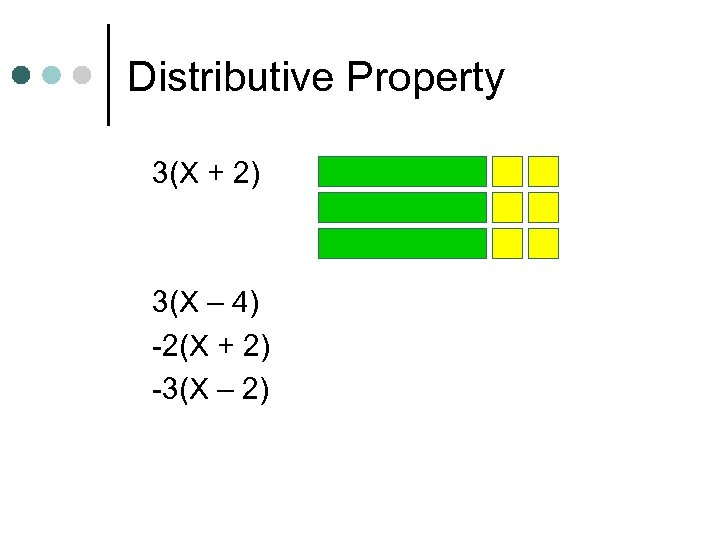Distributive Property 3(X + 2) 3(X – 4) -2(X + 2) -3(X – 2)Multiplication using “base ten blocks. ” (12)(13) ¢ Think of it as (10+2)(10+3) ¢ Multiplication using the array method allows students to see all four subproducts. ¢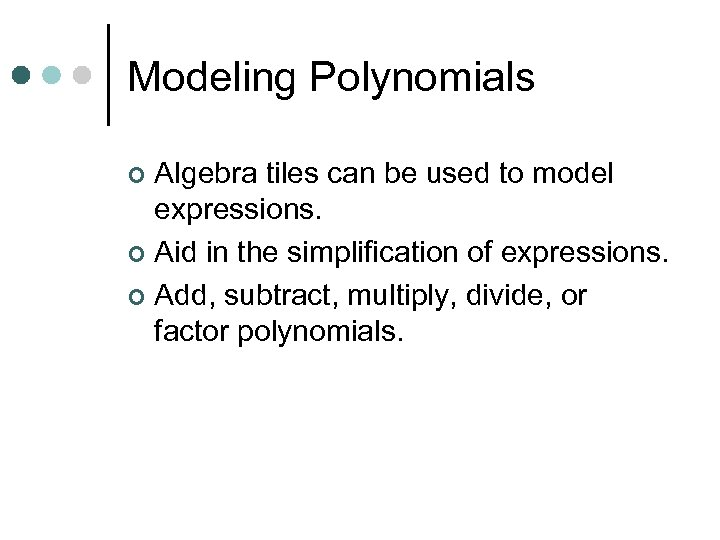Modeling Polynomials Algebra tiles can be used to model expressions. ¢ Aid in the simplification of expressions. ¢ Add, subtract, multiply, divide, or factor polynomials. ¢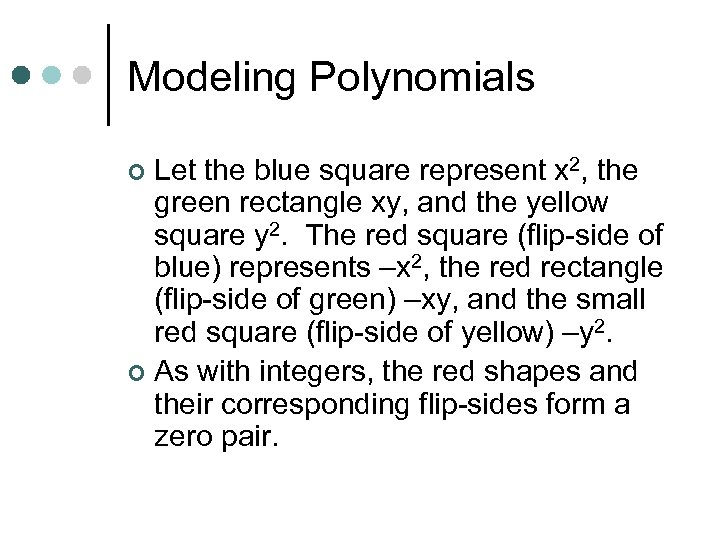Modeling Polynomials Let the blue square represent x 2, the green rectangle xy, and the yellow square y 2. The red square (flip-side of blue) represents –x 2, the red rectangle (flip-side of green) –xy, and the small red square (flip-side of yellow) –y 2. ¢ As with integers, the red shapes and their corresponding flip-sides form a zero pair. ¢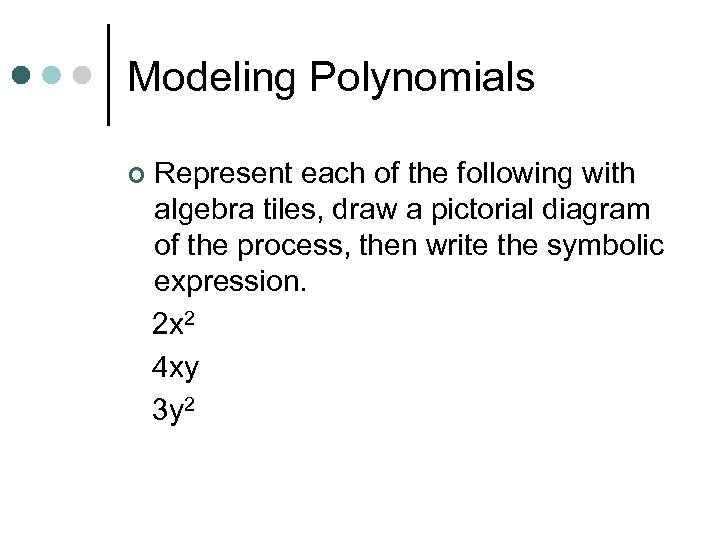Modeling Polynomials ¢ Represent each of the following with algebra tiles, draw a pictorial diagram of the process, then write the symbolic expression. 2 x 2 4 xy 3 y 2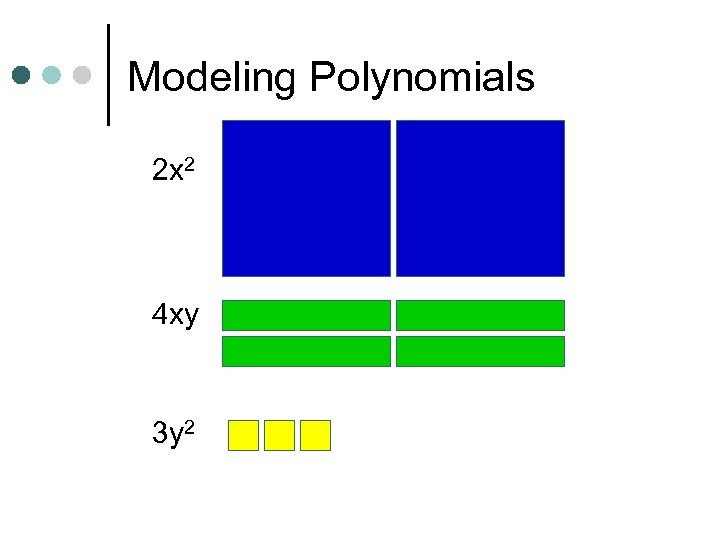Modeling Polynomials 2 x 2 4 xy 3 y 2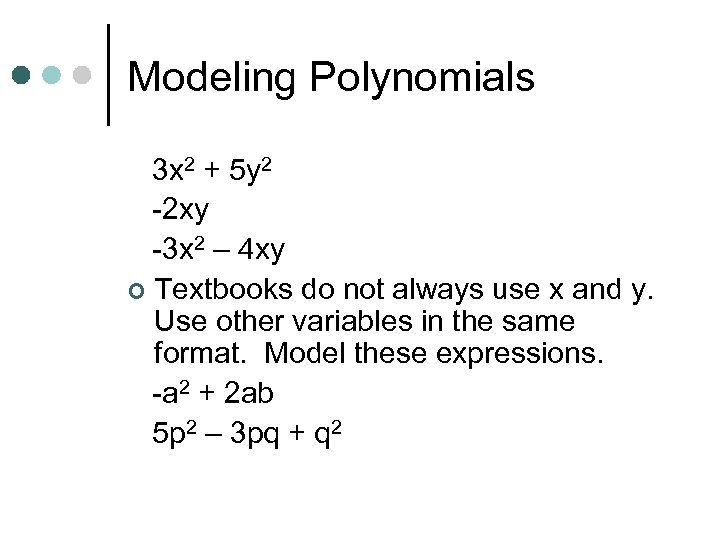Modeling Polynomials 3 x 2 + 5 y 2 -2 xy -3 x 2 – 4 xy ¢ Textbooks do not always use x and y. Use other variables in the same format. Model these expressions. -a 2 + 2 ab 5 p 2 – 3 pq + q 2More Polynomials ¢ ¢ Would not present previous material and this information on the same day. Let the blue square represent x 2 and the large red square (flip-side) be –x 2. Let the green rectangle represent x and the red rectangle (flip-side) represent –x. Let yellow square represent 1 and the small red square (flip-side) represent – 1.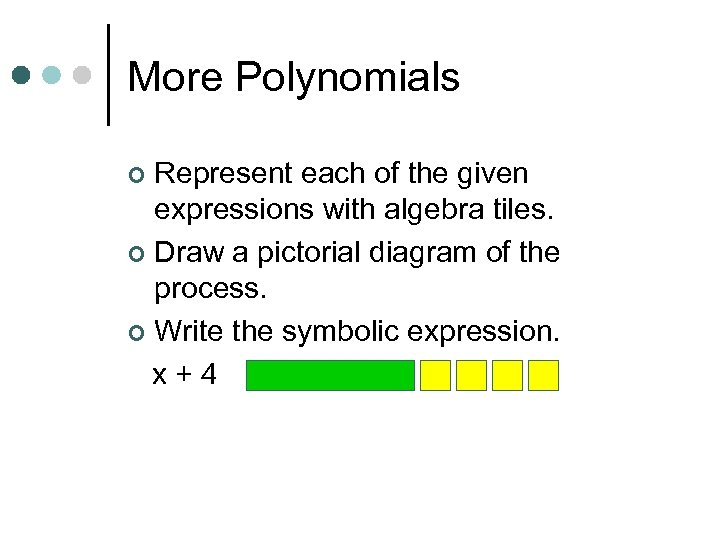More Polynomials Represent each of the given expressions with algebra tiles. ¢ Draw a pictorial diagram of the process. ¢ Write the symbolic expression. x+4 ¢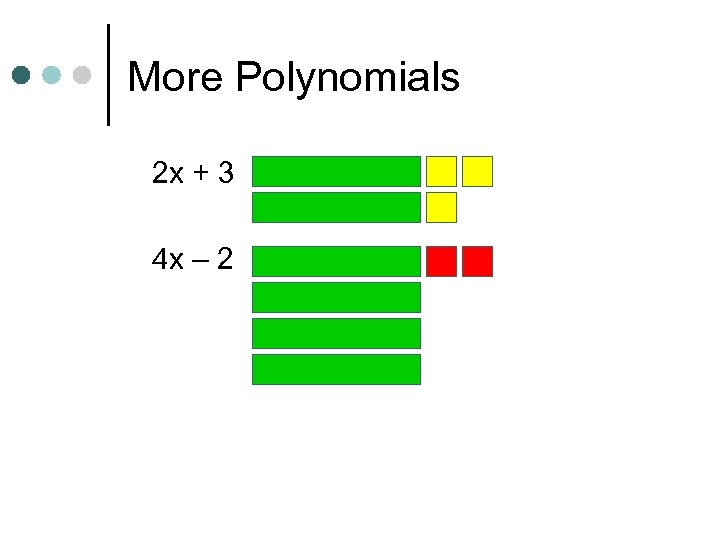More Polynomials 2 x + 3 4 x – 2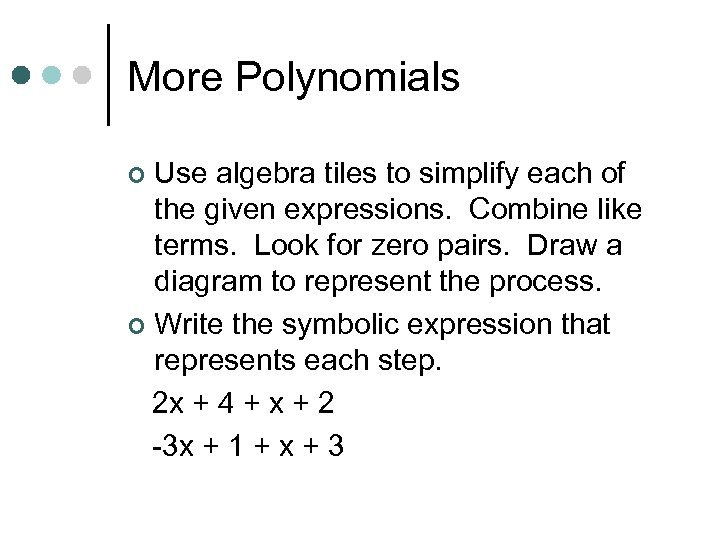More Polynomials Use algebra tiles to simplify each of the given expressions. Combine like terms. Look for zero pairs. Draw a diagram to represent the process. ¢ Write the symbolic expression that represents each step. 2 x + 4 + x + 2 -3 x + 1 + x + 3 ¢More Polynomials 2 x + 4 + x + 2 -3 x + 1 + x + 3More Polynomials 3 x + 1 – 2 x + 4 ¢ This process can be used with problems containing x 2. (2 x 2 + 5 x – 3) + (-x 2 + 2 x + 5) (2 x 2 – 2 x + 3) – (3 x 2 + 3 x – 2)Substitution ¢ Algebra tiles can be used to model substitution. Represent original expression with tiles. Then replace each rectangle with the appropriate tile value. Combine like terms. 3 + 2 x let x = 4Substitution 3 + 2 x let x = 4 3 + 2 x let x = -4 3 – 2 x let x = 4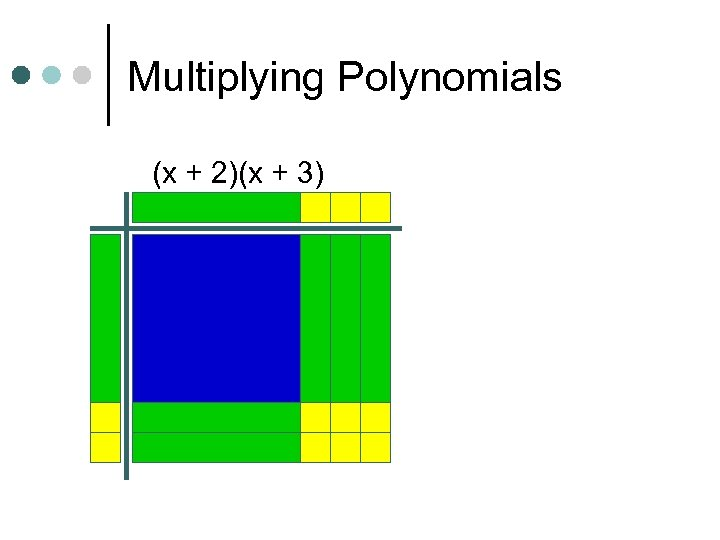Multiplying Polynomials (x + 2)(x + 3)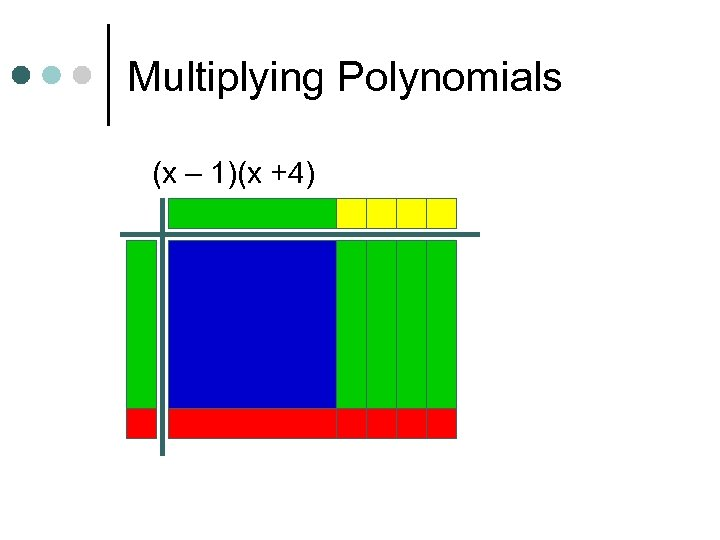Multiplying Polynomials (x – 1)(x +4)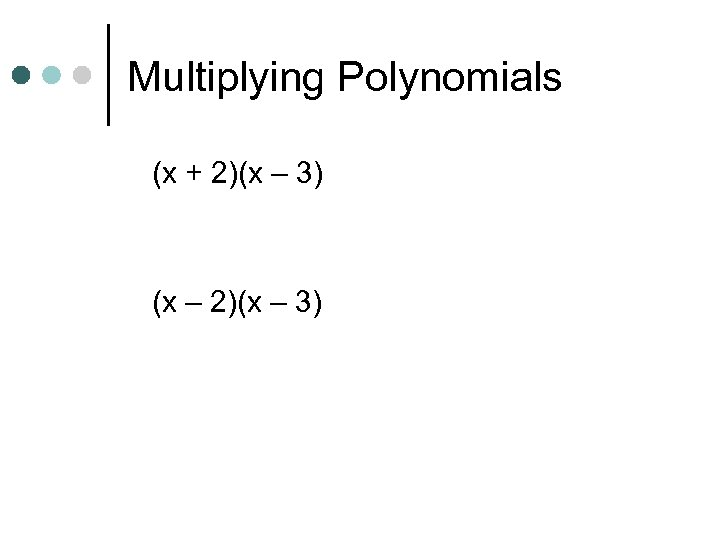Multiplying Polynomials (x + 2)(x – 3) (x – 2)(x – 3)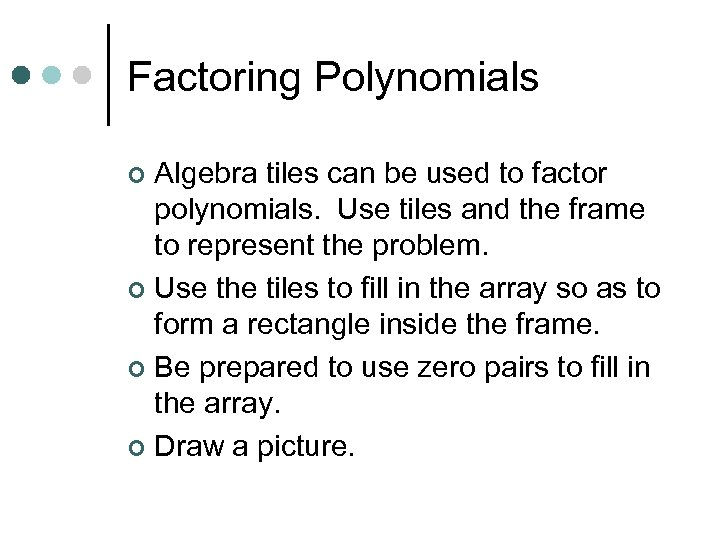Factoring Polynomials Algebra tiles can be used to factor polynomials. Use tiles and the frame to represent the problem. ¢ Use the tiles to fill in the array so as to form a rectangle inside the frame. ¢ Be prepared to use zero pairs to fill in the array. ¢ Draw a picture. ¢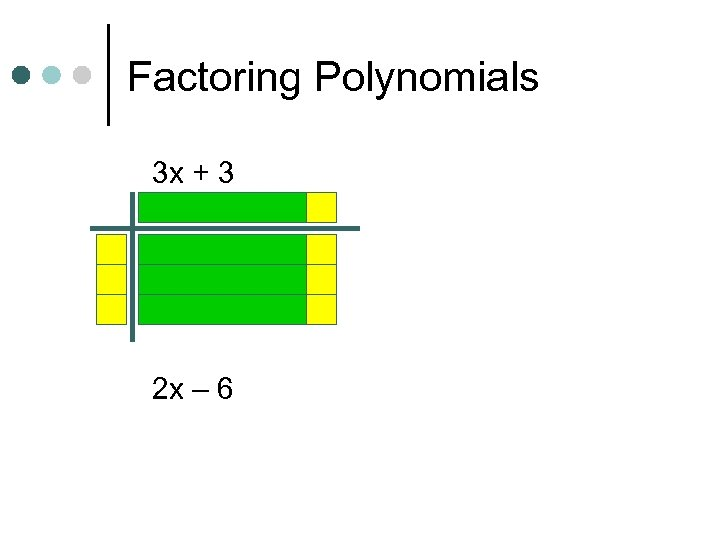Factoring Polynomials 3 x + 3 2 x – 6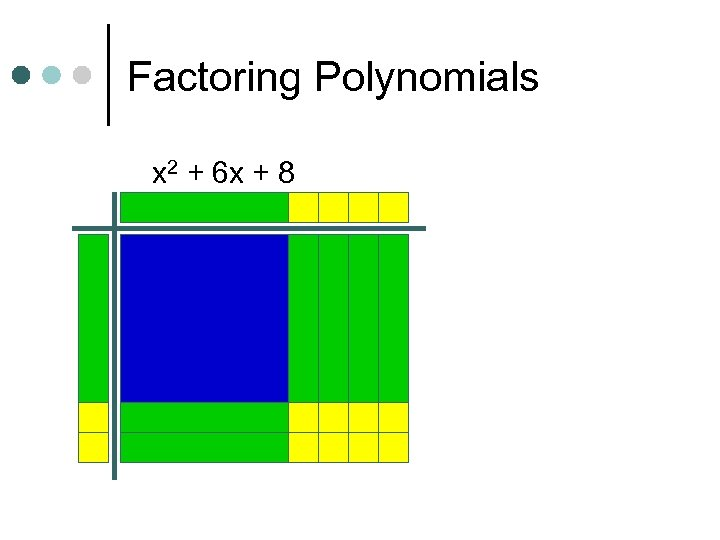Factoring Polynomials x 2 + 6 x + 8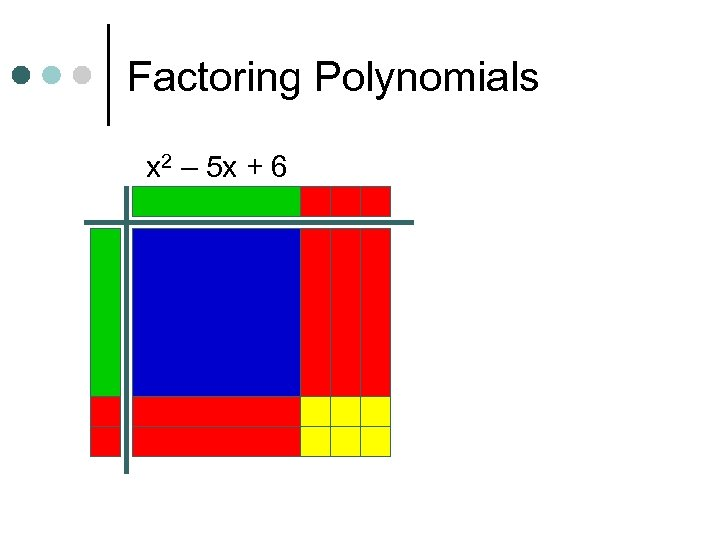Factoring Polynomials x 2 – 5 x + 6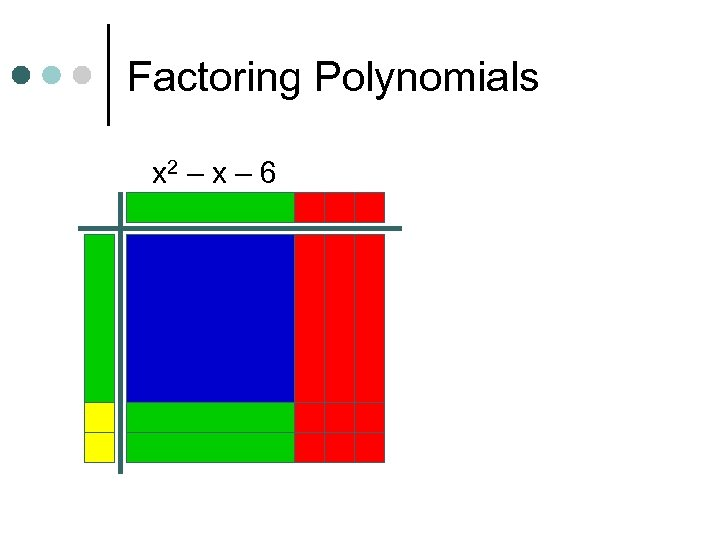Factoring Polynomials x 2 – x – 6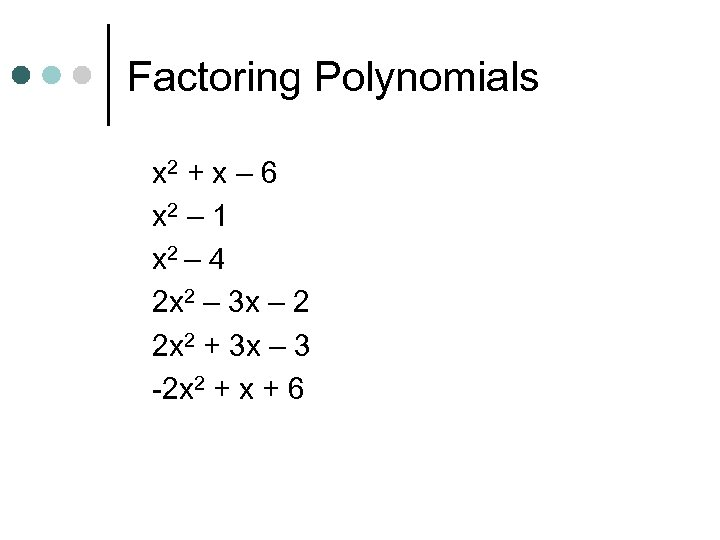Factoring Polynomials x 2 + x – 6 x 2 – 1 x 2 – 4 2 x 2 – 3 x – 2 2 x 2 + 3 x – 3 -2 x 2 + x + 6Dividing Polynomials Algebra tiles can be used to divide polynomials. ¢ Use tiles and frame to represent problem. Dividend should form array inside frame. Divisor will form one of the dimensions (one side) of the frame. ¢ Be prepared to use zero pairs in the dividend. ¢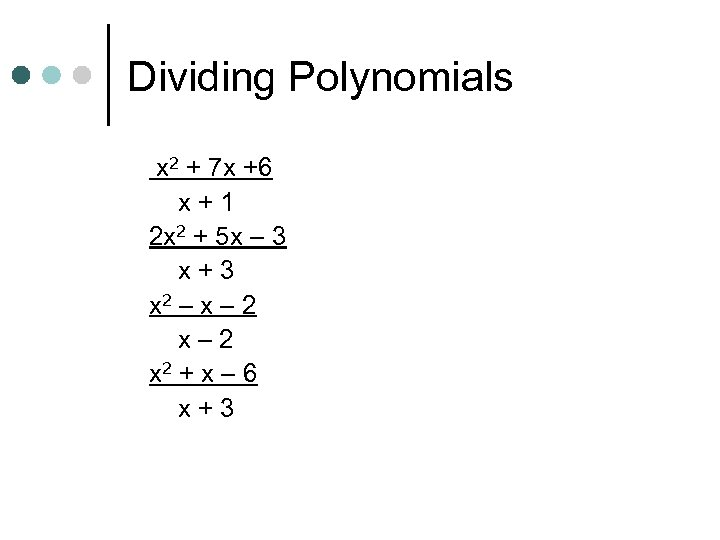Dividing Polynomials x 2 + 7 x +6 x+1 2 x 2 + 5 x – 3 x+3 x 2 – x – 2 x 2 + x – 6 x+3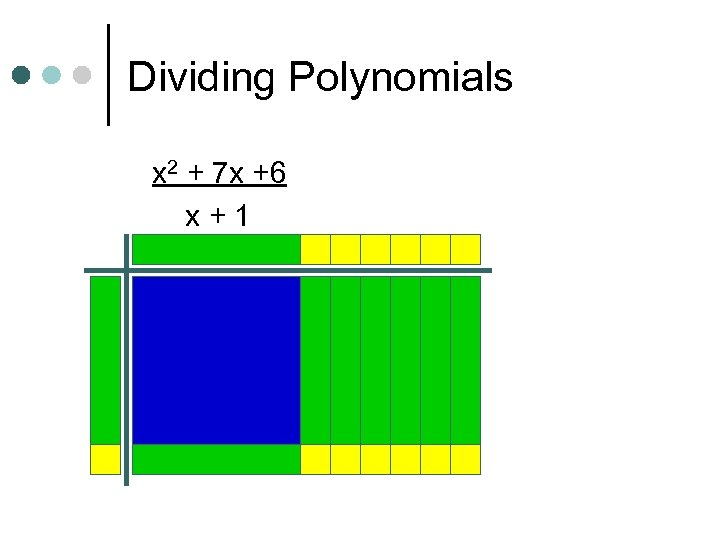Dividing Polynomials x 2 + 7 x +6 x+1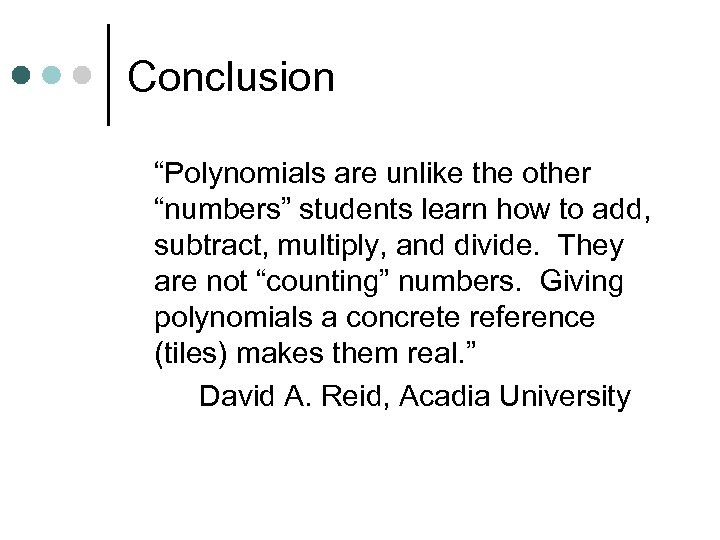Conclusion “Polynomials are unlike the other “numbers” students learn how to add, subtract, multiply, and divide. They are not “counting” numbers. Giving polynomials a concrete reference (tiles) makes them real. ” David A. Reid, Acadia University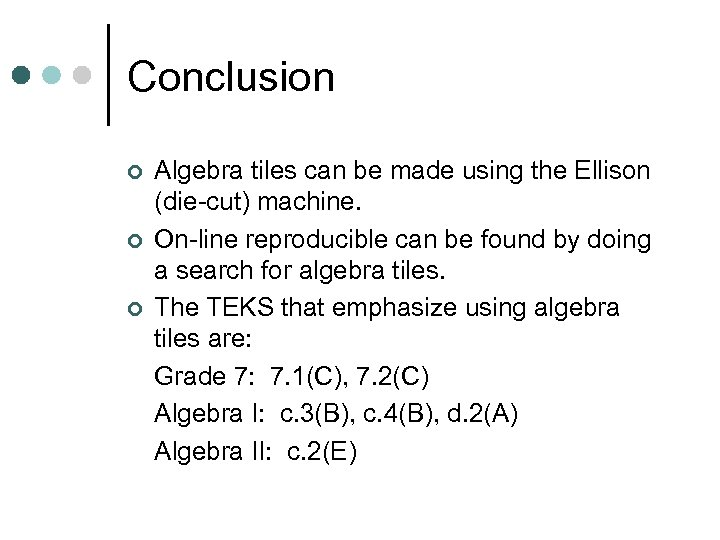Conclusion ¢ ¢ ¢ Algebra tiles can be made using the Ellison (die-cut) machine. On-line reproducible can be found by doing a search for algebra tiles. The TEKS that emphasize using algebra tiles are: Grade 7: 7. 1(C), 7. 2(C) Algebra I: c. 3(B), c. 4(B), d. 2(A) Algebra II: c. 2(E)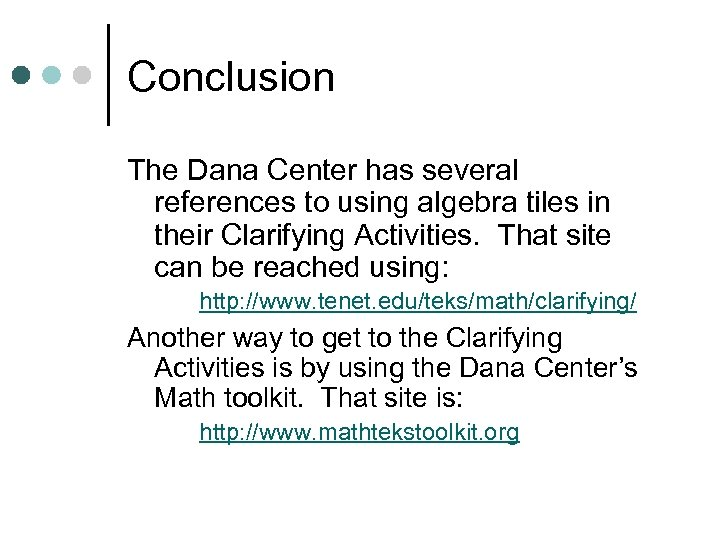Conclusion The Dana Center has several references to using algebra tiles in their Clarifying Activities. That site can be reached using: http: //www. tenet. edu/teks/math/clarifying/ Another way to get to the Clarifying Activities is by using the Dana Center’s Math toolkit. That site is: http: //www. mathtekstoolkit. org